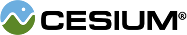#Spherical

#### new Cesium.Spherical(clock, cone, magnitude) Core/Spherical.js 22

A set of curvilinear 3-dimensional coordinates.
Name Type Default Description
`clock` Number `0.0` optional The angular coordinate lying in the xy-plane measured from the positive x-axis and toward the positive y-axis.
`cone` Number `0.0` optional The angular coordinate measured from the positive z-axis and toward the negative z-axis.
`magnitude` Number `1.0` optional The linear coordinate measured from the origin.

### Methods

#### staticCesium.Spherical.clone(spherical, result) → Spherical Core/Spherical.js 64

Creates a duplicate of a Spherical.
Name Type Description
`spherical` Spherical The spherical to clone.
`result` Spherical optional The object to store the result into, if undefined a new instance will be created.
##### Returns:
The modified result parameter or a new instance if result was undefined. (Returns undefined if spherical is undefined)

#### staticCesium.Spherical.equals(left, right) → Boolean Core/Spherical.js 110

Returns true if the first spherical is equal to the second spherical, false otherwise.
Name Type Description
`left` Spherical The first Spherical to be compared.
`right` Spherical The second Spherical to be compared.
##### Returns:
true if the first spherical is equal to the second spherical, false otherwise.

#### staticCesium.Spherical.equalsEpsilon(left, right, epsilon) → Boolean Core/Spherical.js 127

Returns true if the first spherical is within the provided epsilon of the second spherical, false otherwise.
Name Type Default Description
`left` Spherical The first Spherical to be compared.
`right` Spherical The second Spherical to be compared.
`epsilon` Number `0.0` optional The epsilon to compare against.
##### Returns:
true if the first spherical is within the provided epsilon of the second spherical, false otherwise.

#### staticCesium.Spherical.fromCartesian3(cartesian3, spherical) → Spherical Core/Spherical.js 35

Converts the provided Cartesian3 into Spherical coordinates.
Name Type Description
`cartesian3` Cartesian3 The Cartesian3 to be converted to Spherical.
`spherical` Spherical optional The object in which the result will be stored, if undefined a new instance will be created.
##### Returns:
The modified result parameter, or a new instance if one was not provided.

#### staticCesium.Spherical.normalize(spherical, result) → Spherical Core/Spherical.js 86

Computes the normalized version of the provided spherical.
Name Type Description
`spherical` Spherical The spherical to be normalized.
`result` Spherical optional The object to store the result into, if undefined a new instance will be created.
##### Returns:
The modified result parameter or a new instance if result was undefined.

#### clone(result) → Spherical Core/Spherical.js 153

Creates a duplicate of this Spherical.
Name Type Description
`result` Spherical optional The object to store the result into, if undefined a new instance will be created.
##### Returns:
The modified result parameter or a new instance if result was undefined.

#### equals(other) → Boolean Core/Spherical.js 143

Returns true if this spherical is equal to the provided spherical, false otherwise.
Name Type Description
`other` Spherical The Spherical to be compared.
##### Returns:
true if this spherical is equal to the provided spherical, false otherwise.

#### equalsEpsilon(other, epsilon) → Boolean Core/Spherical.js 164

Returns true if this spherical is within the provided epsilon of the provided spherical, false otherwise.
Name Type Description
`other` Spherical The Spherical to be compared.
`epsilon` Number The epsilon to compare against.
##### Returns:
true if this spherical is within the provided epsilon of the provided spherical, false otherwise.

#### toString() → String Core/Spherical.js 173

Returns a string representing this instance in the format (clock, cone, magnitude).
##### Returns:
A string representing this instance.